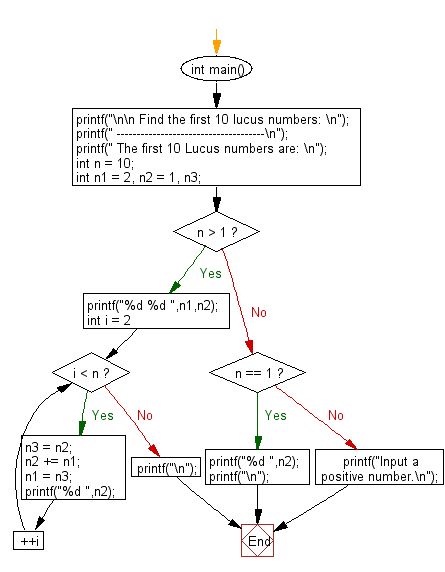﻿ C Program: Display the first 10 lucus numbers - w3resource# C Exercises: Display the first 10 lucus numbers

## C Numbers: Exercise-11 with Solution

Write a program in C to display the first 10 lucus numbers.

Sample Solution:

C Code:

``````# include <stdio.h>
# include <math.h>
# include <stdlib.h>

int main()
{
printf("\n\n Find the first 10 lucus numbers: \n");
printf(" -------------------------------------\n");
printf(" The first 10 Lucus numbers are: \n");

int n = 10;
int n1 = 2, n2 = 1, n3;
if (n > 1)
{
printf("%d %d ",n1,n2);
for(int i = 2; i < n; ++i)
{
n3 = n2;
n2 += n1;
n1 = n3;
printf("%d ",n2);
}
printf("\n");
}
else if (n == 1)
{
printf("%d ",n2);
printf("\n");
}
else
{
printf("Input a positive number.\n");
}
}
```
```

Sample Output:

``` The first 10 Lucus numbers are:
2 1 3 4 7 11 18 29 47 76
```

Flowchart:C Programming Code Editor:

What is the difficulty level of this exercise?

Test your Programming skills with w3resource's quiz.

﻿

## C Programming: Tips of the Day

Why do C and C++ compilers allow array lengths in function signatures when they're never enforced?

It is a quirk of the syntax for passing arrays to functions.

Actually it is not possible to pass an array in C. If you write syntax that looks like it should pass the array, what actually happens is that a pointer to the first element of the array is passed instead.

Since the pointer does not include any length information, the contents of your [] in the function formal parameter list are actually ignored.

Ref : https://bit.ly/3fhlvdH